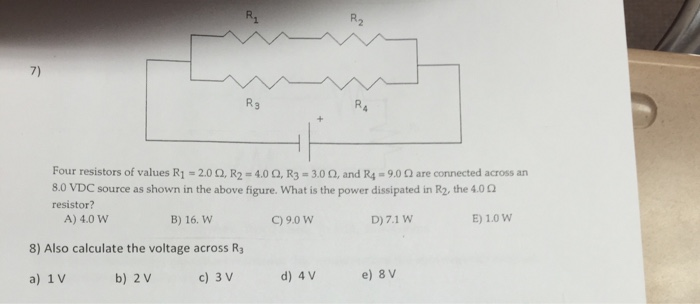# Rc circuit R2 7) R3 Four resistors of values R1 = 2.0 Ω, R2 = 4.0...

###### Question:

Rc circuitR2 7) R3 Four resistors of values R1 = 2.0 Ω, R2 = 4.0 Ω, R3-3.00, and R4° 9.0 Ω are connected across an 8.0 VDC source as shown in the above figure, what is the power dissipated in R2, the 4.00 resistor? A) 4.0 W B) 16. W C)9.0 W D) 7.1 W E) 1.0 W 8) Also calculate the voltage across R a) 1 V b) 2 V c) 3 V d) 4 V